Author: Oscar Cronquist Article last updated on April 14, 2021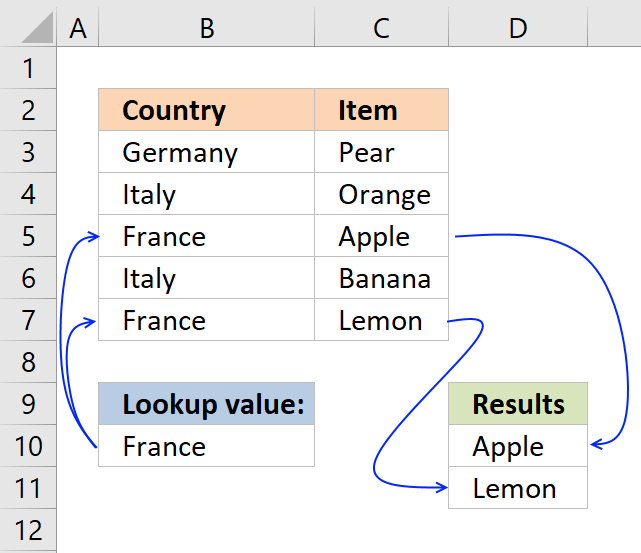The VLOOKUP function is designed to return only a corresponding value of the first instance of a lookup value, from a column you choose. But there is a workaround to identify multiple matches.

The array formulas demonstrated below are smaller and easier to understand and troubleshoot than the useful VLOOKUP function.

However you are not limited to array formulas, Excel also has built-in features that work very well, you will be amazed at how easy it is to filter values in a data set.

I have made a formula, demonstrated in a separate article, that allows you to VLOOKUP and return multiple values across worksheets, there is also an Add-In that makes it even easier to accomplish this task.

Now, if you only need one instance of each returned value then check this article out: Vlookup – Return multiple unique distinct values It lets you specify a condition and the formula is not even an array formula.

I have also written an article about searching for a string (wildcard search) and return corresponding values, it requires a somewhat more complicated formula but don't worry, you will find an explanation there, as well.

Did you know that it is also possible to VLOOKUP and return multiple values distributed over several columns, the formula even ignores blanks.

## 1. VLOOKUP - Return multiple values verticallyCan VLOOKUP return multiple values? It can, however the formula would become huge if it needs to contain the VLOOKUP function. The formula presented here does not contain that function, however, it is more versatile and smaller.

The image above shows you an array formula that extracts adjacent values based on a lookup value in cell D10.

Another great thing with this array formula is that it allows you to lookup and return values from whatever column you like contrary to the VLOOKUP function that lets you only do a lookup in the left-most column, in a given range.

Update 17 December 2020, check out the new FILTER function available for Excel 365 users. Regular formula in cell D10:

=FILTER(C3:C7, B10=B3:B\$7)

Read here about how it works: Filter values based on a condition

The following formula is for earlier Excel versions. Array formula in D10:

=INDEX(\$C\$3:\$C\$7, SMALL(IF((\$B\$10=\$B\$3:\$B\$7), MATCH(ROW(\$B\$3:\$B\$7), ROW(\$B\$3:\$B\$7)), ""),ROWS(\$A\$1:A1)))

This video explains how to VLOOKUP and return multiple matching values:

The array formula in cell G3 looks in column B for "France" and return adjacent values from column C. The array formula in cell G3 filters values unsorted, if you want to sort returning values alphabetically, read this:
Vlookup with 2 or more lookup criteria and return multiple matches

### How to create an array formula

1. Copy array formula above. (Ctrl + c)
2. Double-press with left mouse button on a cell.
3. Paste (Ctrl + v) array formula.
4. Press and hold Ctrl + Shift simultaneously.
5. Press Enter once.
6. Release all keys.

The array formula above filters only values with one condition, the following article explains how to filter based on multiple criteria: Vlookup with 2 or more lookup criteria and return multiple matches

If you don't like array formulas, try this regular but more complicated formula in cell D10:

=INDEX(\$C\$3:\$C\$7,SMALL(INDEX((\$B\$10=\$B\$3:\$B\$7)*(MATCH(ROW(\$B\$3:\$B\$7), ROW(\$B\$3:\$B\$7)))+(\$E\$3<>\$B\$3:\$B\$7)*1048577,),ROWS(\$A\$1:A1)))

### Explaining array formula (Return values vertically)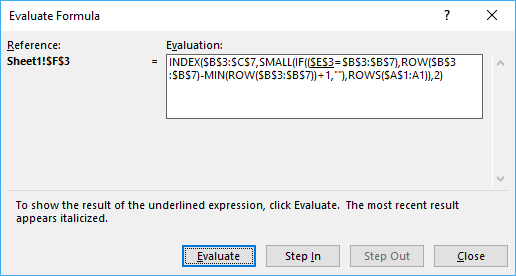You can easily follow along as I explain the formula, select cell D10. Go to tab "Formulas" on the ribbon and press with left mouse button on "Evaluate Formula" button. Press with left mouse button on "Evaluate" button shown above to move to next step.

#### Step 1 - Identify cells equal to the condition in cell B10

= (equal sign) is a comparison operator and checks if criterion (E3) is equal to values in array (\$B\$3:\$B\$7). This operator is not case sensitive.

\$B\$10=\$B\$3:\$B\$7

becomes

"France"={"Germany";"Italy";"France";"Italy";"France"}

and returns

{FALSE, FALSE, TRUE, FALSE, TRUE}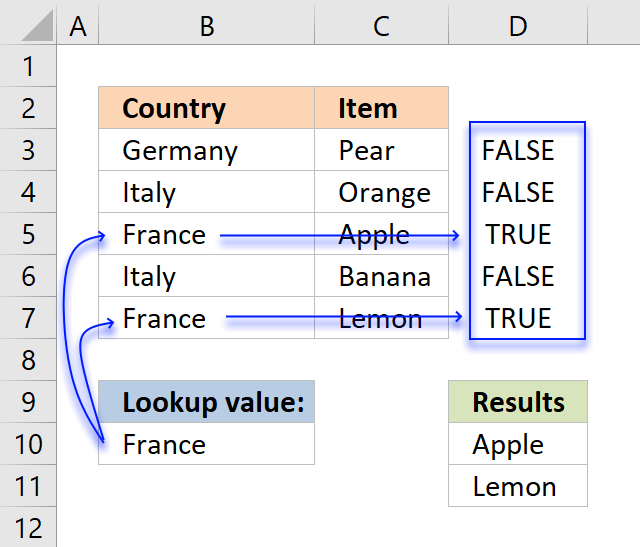The image above shows an array in cell range D3:D7 containing boolean values, those values correspond to the logical expression if cell B10 is equal B3:B7. Cell B5 and B7 is equal to cell B10, these return TRUE. The other remaining cells is not equal to cell B10 and return FALSE.

#### Step 2 - Create array containing corresponding row numbers

The ROW function returns the row number based on a cell reference, we are using a cell reference that points to a cell range containing multiple rows so the ROW function returns an array of row numbers.

MATCH(ROW(\$B\$3:\$B\$7), ROW(\$B\$3:\$B\$7))

The MATCH function finds the relative position of a value in a cell range or array, however, I am using multiple values so this step returns an array of numbers.

MATCH(ROW(\$B\$3:\$B\$7), ROW(\$B\$3:\$B\$7))

becomes

MATCH({3, 4, 5, 6, 7}, {3, 4, 5, 6, 7})

and returns {1,2,3,4,5}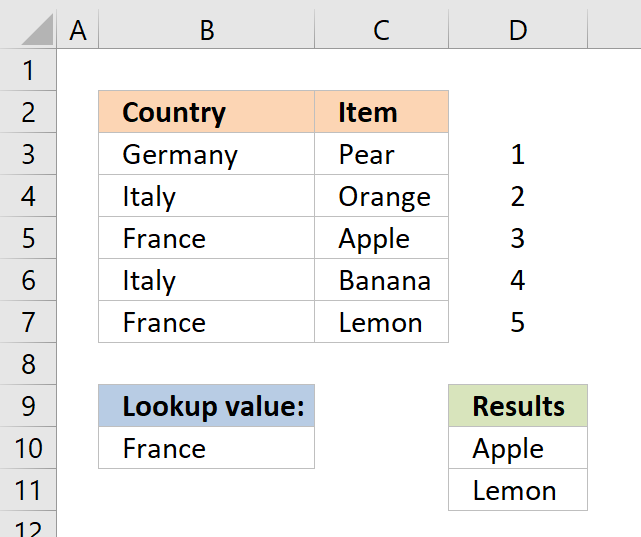The image above shows the array in cell range D3:D7, the array always begins with 1 and has must have the same number of values in the array as the table has rows.

#### Step 3 - Filter row numbers equal to a condition

The IF function has three arguments, the first one must be a logical expression. If the expression evaluates to TRUE then one thing happens (argument 2) and if FALSE another thing happens (argument 3).

IF((\$B\$10=\$B\$3:\$B\$7), MATCH(ROW(\$B\$3:\$B\$7), ROW(\$B\$3:\$B\$7)), "")

becomes

IF(FALSE, FALSE, TRUE, FALSE, TRUE}, MATCH(ROW(\$B\$3:\$B\$7), ROW(\$B\$3:\$B\$7)), "")

becomes

IF(FALSE, FALSE, TRUE, FALSE, TRUE},{1, 2, 3, 4, 5}, "")

and returns {"", "", 3, "", 5}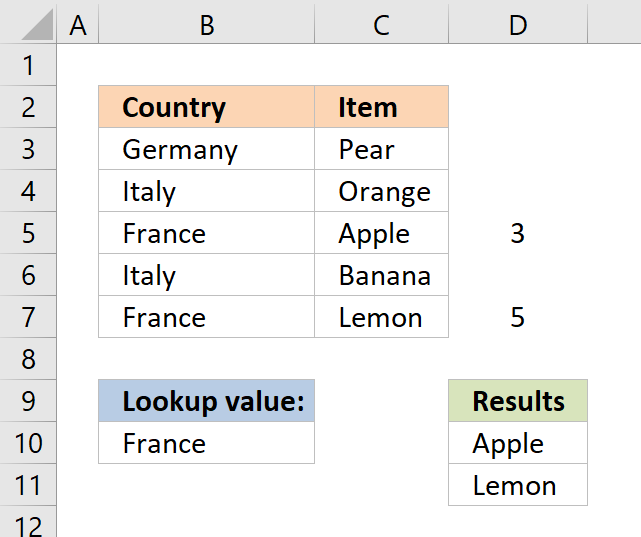The IF function replaces the numbers that correspond to boolean value FALSE with "" (nothing) and boolean value TRUE with a number, shown in cell range D3:D7.

#### Step 4 - Return the k-th smallest row number

To be able to return a new value in a cell each I use the SMALL function to filter row numbers from smallest to largest.

The ROWS function keeps track of the numbers based on an expanding cell reference. It will expand as the formula is copied to the cells below.

SMALL(IF((\$E\$3=\$B\$3:\$B\$7),ROW(\$B\$3:\$B\$7)-MIN(ROW(\$B\$3:\$B\$7))+1,""),ROWS(\$A\$1:A1))

becomes

SMALL({"", "", 3, "", 5}, ROWS(\$A\$1:A1))

This part of the formula returns the k-th smallest number in the array {"", "", 3, "", 5}

To calcualte the k-th smallest value I am using ROWS(\$A\$1:A1) to create the number 1.

When the formula in cell D10 is copied to cell D11, ROWS(\$A\$1:A1) changes to ROWS(\$A\$1:A2). ROWS(\$A\$1:A2) returns 2.

In Cell D10: =INDEX(\$C\$3:\$C\$7, SMALL({"", "", 3, "", 5}, ROWS(\$A\$1:A1))

=INDEX(\$C\$3:\$C\$7, SMALL({"", "", 3, "", 5}, 1))

The smallest number in array {"", "", 3, "", 5} is 3.

In Cell D11: =INDEX(\$C\$3:\$C\$7, SMALL({"", "", 3, "", 5}, ROWS(\$A\$1:A2)))

=INDEX(\$C\$3:\$C\$7, SMALL({"", "", 3, "", 5}, 2))

The second smallest number in array {"", "", 3, "", 5} is 5.

#### Step 4 - Return value based on row number

The INDEX function returns a value based on a cell reference and column/row numbers.

In Cell D10:

=INDEX(\$C\$3:\$C\$7, 3)

becomes

=INDEX({"Pear", "Orange", "Apple", "Banana", "Lemon"}, 3)

and returns "Apple" in cell D10.

In Cell D11:

=INDEX(\$C\$3:\$C\$7, 5) returns "Lemon"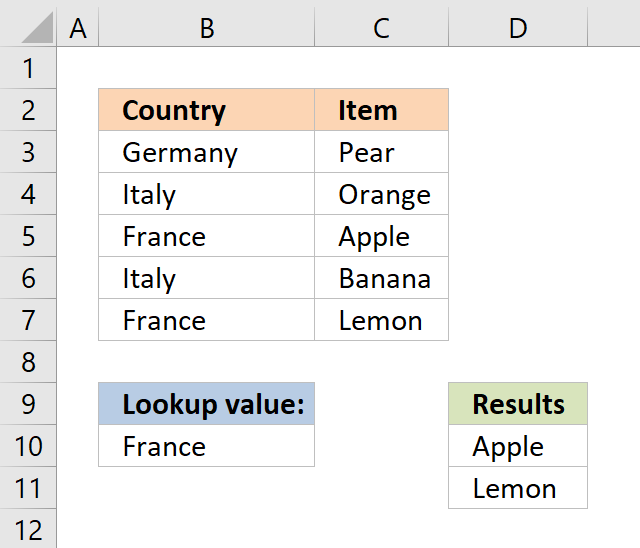This article demonstrates how to filter an Excel defined table programmatically based on a condition using event code and a macro.

### Get the Excel fileHow-to-return-multiple-values-vertically-2.xlsx

## 1.1 Return multiple values - case sensitive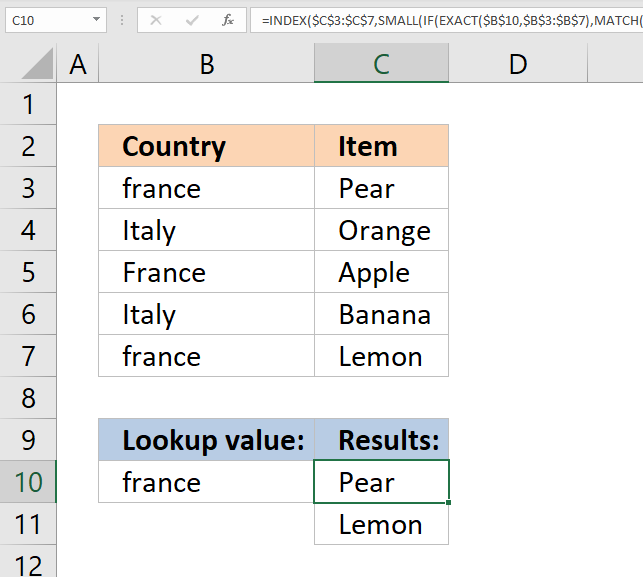The image above demonstrates a formula in cell C10 that extracts values from cell range C3:C7 if the corresponding value in cell range B3:B7 is equal to the value in cell B10.

The values in cell B3:B7  must have the same upper and lower letters as the lookup value in cell B10 to generate a match.

Lookup value "france" is found in cell B3 and B7 but not in cell B5. Cell B5 has a value that begins with an upper letter. The corresponding cells to B3 and B7 are C3 and C7, those values are returned in cell C10 and cells below.

Array formula in cell C10:

=INDEX(\$C\$3:\$C\$7, SMALL(IF(EXACT(\$B\$10, \$B\$3:\$B\$7), MATCH(ROW(\$B\$3:\$B\$7), ROW(\$B\$3:\$B\$7)), ""), ROWS(\$A\$1:A1)))

How to enter an array formula

Copy cell C10 and paste to cells below.

If you own Excel 365 you can use the much easier FILTER function to accomplish the same thing: Filter values based on a condition - case sensitive

## 1.2 Return multiple values - if not equal to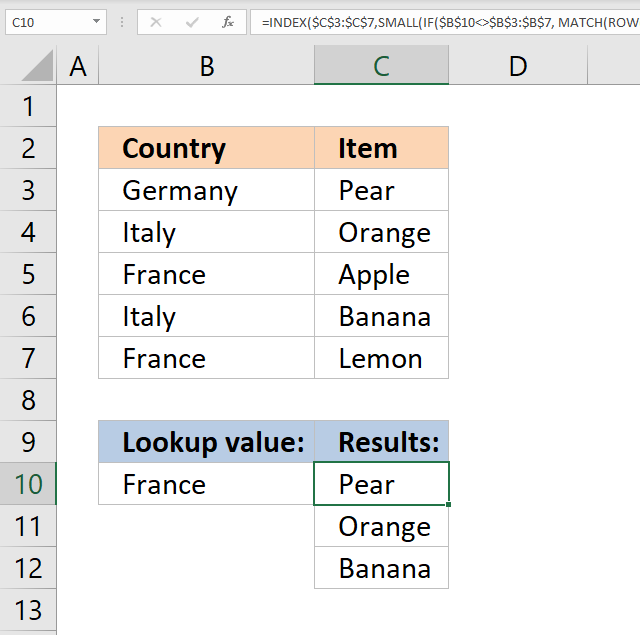The picture above shows an array formula in cell C10 that extracts values from cell range C3:C7 if the corresponding value in cell range B3:B7 is NOT equal to the lookup value in cell B10.

The lookup value in cell B10 is not equal to the value in B3, B4, and B6. The corresponding values in C3, C4, and C6 are returned to cell C10 and cells below.

Array formula in cell C10:

=INDEX(\$C\$3:\$C\$7, SMALL(IF(\$B\$10<>\$B\$3:\$B\$7, MATCH(ROW(\$B\$3:\$B\$7), ROW(\$B\$3:\$B\$7)), ""), ROWS(\$A\$1:A1)))

How to enter an array formula

Copy cell C10 and paste to cells below.

If you own Excel 365 you can use the much easier FILTER function to accomplish the same thing: Filter values if not equal to

## 1.3 Return multiple values - if smaller than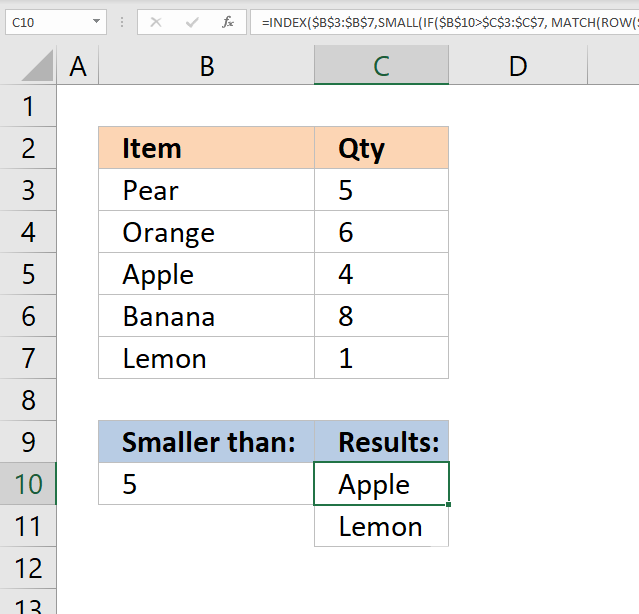The image above demonstrates a formula in cell C10 that extracts items from cell range B3:B7 if the corresponding value in cell range C3:C7 is smaller than the value in cell B10.

In the example above, cells C5 and C7 are smaller than the value in cell B10. The corresponding cells are B5 and B7 which the formula returns in cell C10 and cells below.

Array formula in cell C10:

=INDEX(\$B\$3:\$B\$7, SMALL(IF(\$B\$10>\$C\$3:\$C\$7, MATCH(ROW(\$B\$3:\$B\$7), ROW(\$B\$3:\$B\$7)), ""), ROWS(\$A\$1:A1)))

How to enter an array formula

Copy cell C10 and paste to cells below.

If you own Excel 365 you can use the much easier FILTER function to accomplish the same thing: Filter values if smaller than

## 1.4 Return multiple values - if larger than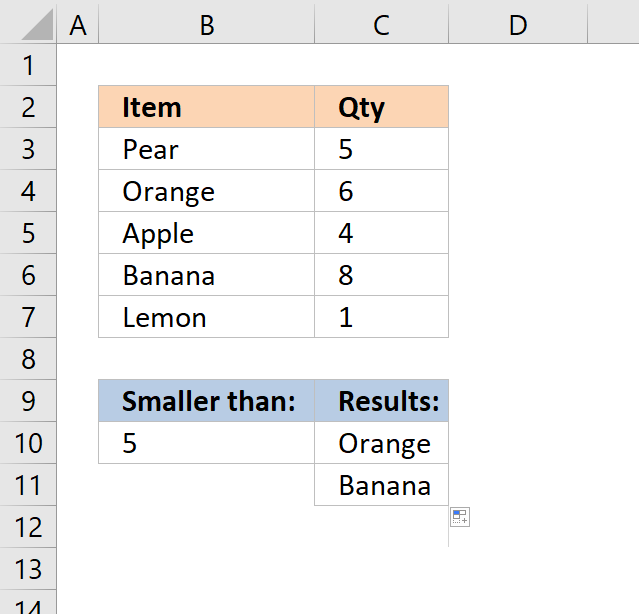The picture above demonstrates a formula in cell C10 that extracts values from cell range B3:B7 if the corresponding values in C3:C7 are less than the value in cell B10.

In this example, cells C5 and C7 are smaller than the value in cell B10. The formula in cell C10 returns the corresponding values from B5 and B7.

Array formula in cell C10:

=INDEX(\$B\$3:\$B\$7, SMALL(IF(\$B\$10<\$C\$3:\$C\$7, MATCH(ROW(\$B\$3:\$B\$7), ROW(\$B\$3:\$B\$7)), ""), ROWS(\$A\$1:A1)))

How to enter an array formula

Copy cell C10 and paste to cells below.

If you own Excel 365 you can use the much easier FILTER function to accomplish the same thing: Filter values if smaller than

## 1.5 Return multiple values - if contains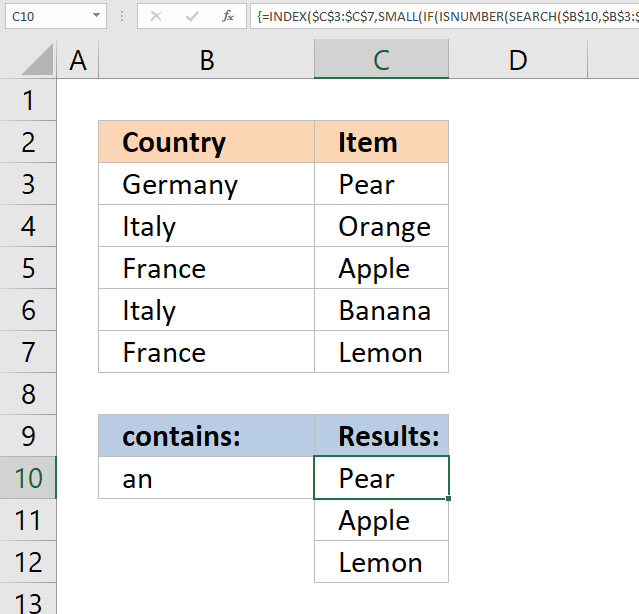The image above demonstrates a formula in cell C10 that extracts values from cell range C3:C7 if the corresponding values in cell range B3:B7 contain the value in cell B10.

In this example, the values in cells B3, B5, and B7 contain the value in cell B10. The array formula returns the corresponding values from cells C3, C5, and C7 to cell C10 and cells below as far as necessary.

Array formula in cell C10:

=INDEX(\$C\$3:\$C\$7, SMALL(IF(ISNUMBER(SEARCH(\$B\$10, \$B\$3:\$B\$7)), MATCH(ROW(\$B\$3:\$B\$7), ROW(\$B\$3:\$B\$7)), ""), ROWS(\$A\$1:A1)))

How to enter an array formula

Copy cell C10 and paste to cells below.

If you own Excel 365 you can use the much easier FILTER function to accomplish the same thing: Filter values if contains

## 1.6 Return multiple values - if not contains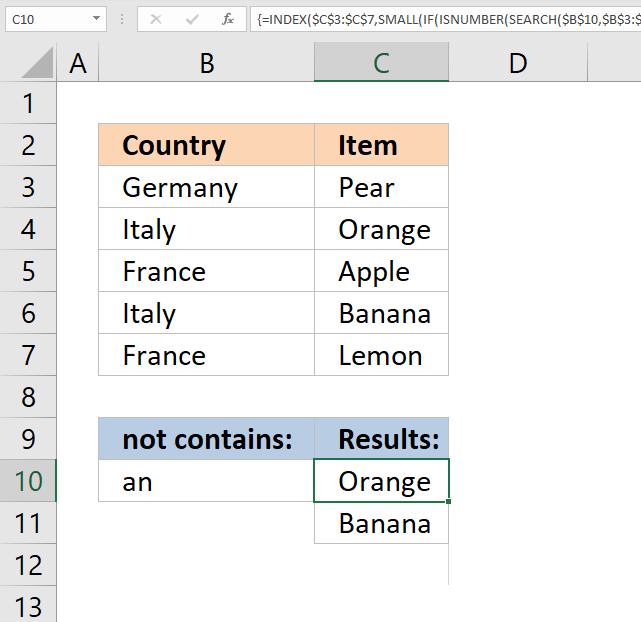The picture above demonstrates a formula in cell C10 that extracts values from cell range C3:C7 if the corresponding values in cell range B3:B7 do not contain the value in cell B10.

In this example, the values in cells B4, and B6 do not contain the value in cell B10. The array formula returns the corresponding values from cells C4, and C6 to cell C10 and cells below as far as necessary.

Array formula in cell C10:

=INDEX(\$C\$3:\$C\$7, SMALL(IF(ISNUMBER(SEARCH(\$B\$10, \$B\$3:\$B\$7)), "", MATCH(ROW(\$B\$3:\$B\$7), ROW(\$B\$3:\$B\$7))), ROWS(\$A\$1:A1)))

How to enter an array formula

Copy cell C10 and paste to cells below.

If you own Excel 365 you can use the much easier FILTER function to accomplish the same thing: Filter values if contains

## 1.7 Return multiple values - that begins with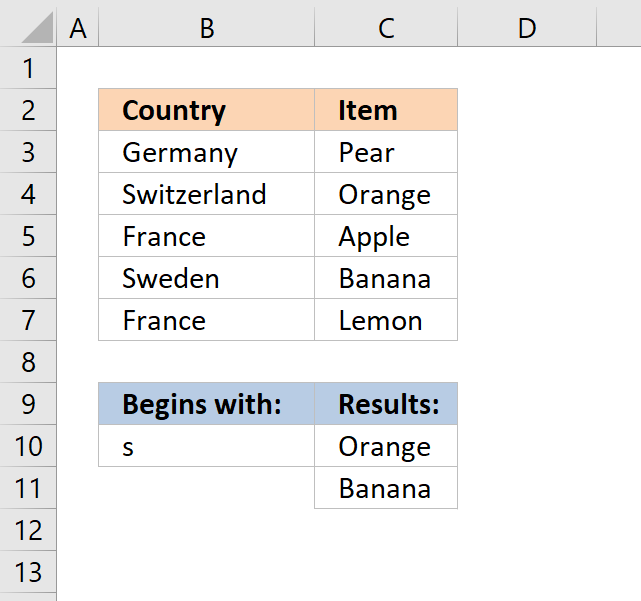The formula in cell C10 extracts values from cell range C3:C7 if the corresponding values in B3:B7 begin with the same value as the value entered in cell B10.

The image above shows that cell B4 and B6 begins with the same value as the value in cell B10. The corresponding values in cell C4 and C6 are displayed in cell C10 and C11.

Array formula in cell C10:

=INDEX(\$C\$3:\$C\$7, SMALL(IF(\$B\$10=LEFT(\$B\$3:\$B\$7, LEN(\$B\$10)), MATCH(ROW(\$B\$3:\$B\$7), ROW(\$B\$3:\$B\$7)), ""), ROWS(\$A\$1:A1)))

How to enter an array formula

Copy cell C10 and paste to cells below as far as needed.

If you own Excel 365 you can use the much easier FILTER function to accomplish the same thing: Filter values that begin with

## 1.8 Return multiple values - that ends with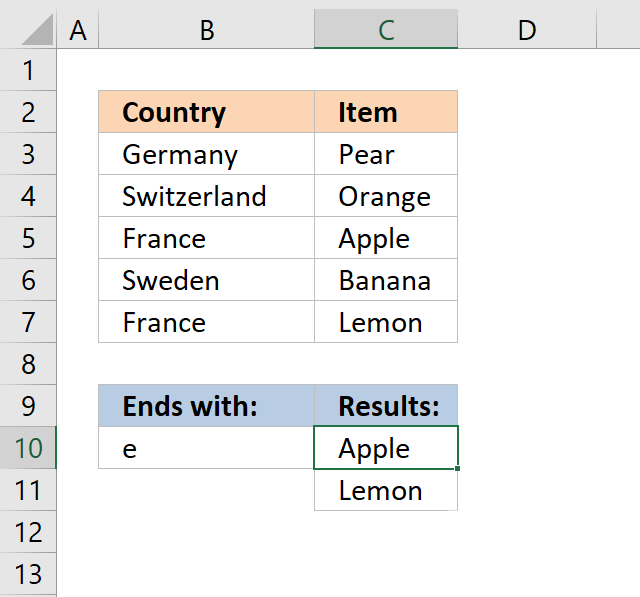Array formula in cell C10:

=INDEX(\$C\$3:\$C\$7, SMALL(IF(RIGHT(\$B\$3:\$B\$7, LEN(\$B\$10))=\$B\$10, MATCH(ROW(\$B\$3:\$B\$7), ROW(\$B\$3:\$B\$7)), ""), ROWS(\$A\$1:A1)))

How to enter an array formula

Copy cell C10 and paste to cells below as far as needed.

If you own Excel 365 you can use the much easier FILTER function to accomplish the same thing: Filter values that end with

## How to remove #num errorsThe picture above shows you the array formula copied down to cell D12 however there are only two values shown, the remaining cells show nothing not even an error.

Array formula in cell D10:

=IFERROR(INDEX(\$C\$3:\$C\$7, SMALL(IF((\$B\$10=\$B\$3:\$B\$7), MATCH(ROW(\$B\$3:\$B\$7), ROW(\$B\$3:\$B\$7)), ""),ROWS(\$A\$1:A1))), "")

The IFERROR function lets you convert error values to blank cells or really in whatever value you want. In this case it returns blank cells.

Note: The IFERROR catches all kinds of formula errors. you won't spot the error that easily if there is some kind of other error in the formula. Use this function with caution.

How to enter an array formula

## Count matching values

The following image shows you a data set in column B and C. The lookup value in cell E3 is used for identifying matching cell values in column B.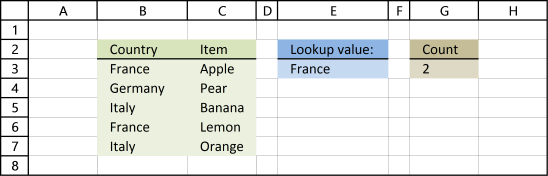Formula in cell G3:

=COUNTIF(\$B\$3:\$B\$7,E3)

Alternative formula in cell G3:

=SUMPRODUCT((\$B\$3:\$B\$7=E3)*1)

## 2 Return multiple values horizontally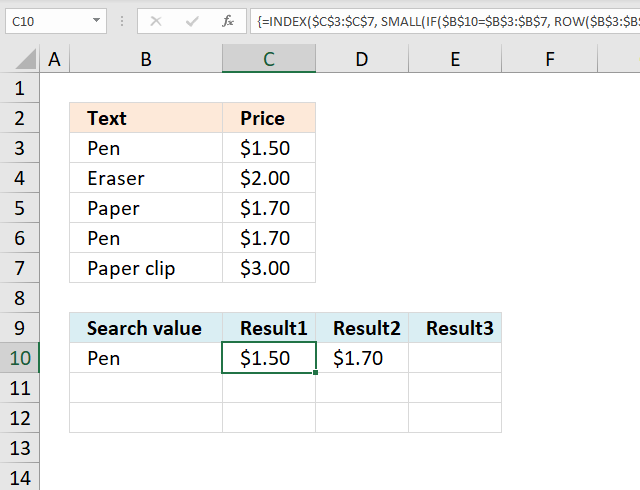Update 17 December 2020, use the FILTER function to return multiple values horizontally. Regular formula in cell C10:

=TRANSPOSE(FILTER(C3:C7, B10=B3:B7))

The formula above works only in Excel 365. The array formula below is for earlier Excel versions and is entered in cell C10.

Array formula in C10:

=INDEX(\$C\$3:\$C\$7, SMALL(IF(\$B\$10=\$B\$3:\$B\$7, ROW(\$B\$3:\$B\$7)-MIN(ROW(\$B\$3:\$B\$7))+1, ""), COLUMNS(\$A\$1:A1)))

Copy cell C10 and paste to cells to the right of cell C10 as far as needed.

Enter the formula above  as an array formula or use this regular but more complicated formula:

=INDEX(\$C\$3:\$C\$7, SMALL(INDEX((\$B\$10=\$B\$3:\$B\$7)*(MATCH(ROW(\$B\$3:\$B\$7), ROW(\$B\$3:\$B\$7))+(\$B\$19<>\$B\$3:\$B\$7)*1048577, 0, 0), COLUMNS(\$A\$1:A1)))

### Get the Excel fileReturn-multiple-values-horizontallyv3.xls

## Extract multiple records based on a condition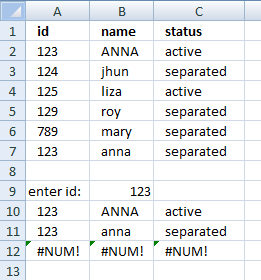Update 17 December 2020, use the new FILTER function to extract values based on a condition, formula in cell A10:

=FILTER(A2:C7, B9=A2:A7)

The FILTER function is available for Excel 365 users and the formula above is entered as a regular formula.

The formula below is for earlier Excel versions, it extracts records based on the value in cell B9. Array formula in cell A10:

=INDEX(\$A\$2:\$C\$7, SMALL(IF(\$B\$9=\$A\$2:\$A\$7, ROW(\$A\$2:\$A\$7)-MIN(ROW(\$A\$2:\$A\$7))+1, ""), ROW(A1)),COLUMN(A1))

To enter an array formula, type the formula in a cell then press and hold CTRL + SHIFT simultaneously, now press Enter once. Release all keys.

The formula bar now shows the formula with a beginning and ending curly bracket telling you that you entered the formula successfully. Don't enter the curly brackets yourself.

Copy cell A10 and paste to cell range B10:C10. Then copy A10:C10 and paste to cell range A11:C12.

#### Watch a video where I explain how to use the array formula and how it works

Enter the formula above as an array formula or use this regular but more complicated formula:

=INDEX(\$A\$2:\$C\$7, SMALL(INDEX((\$B\$9=\$A\$2:\$A\$7)*(MATCH(ROW(\$A\$2:\$A\$7), ROW(\$A\$2:\$A\$7)))+(\$B\$9<>\$A\$2:\$A\$7)*1048577, 0, 0),ROW(A1)),COLUMN(A1))

### Get the Excel fileVlookup-return-multiple-records.xlsx

## Lookup and return multiple values [AutoFilter]

The AutoFilter is a built-in feature in Excel that allows you to quickly filter data. The following video shows you how to quickly filter a data set, I don't think you can do it more quickly than this.

### Instructions on how to filter a data set [AutoFilter]

1. Press with right mouse button on on a cell value that you want to filter
2. Press with mouse on "Filter" and then "Filter by Selected Cell's Value"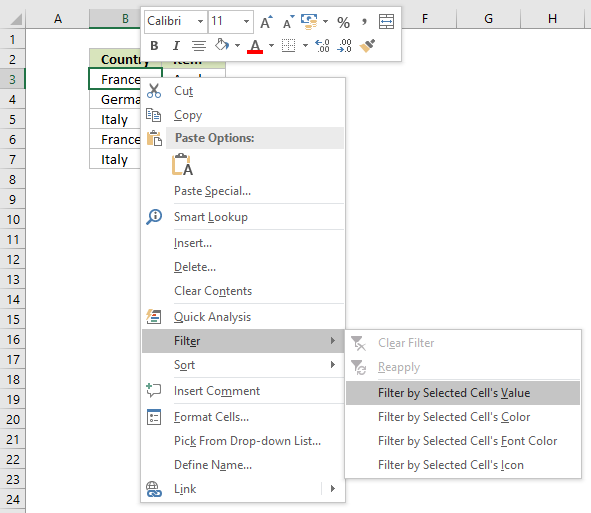3. That's it!

### How to remove a filter

1. Press with mouse on filter button next to header, shown in picture below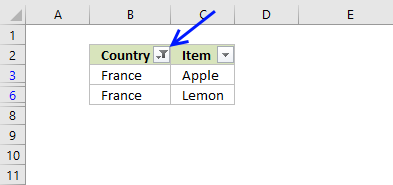2. Press with mouse on "Clear Filter From "Country""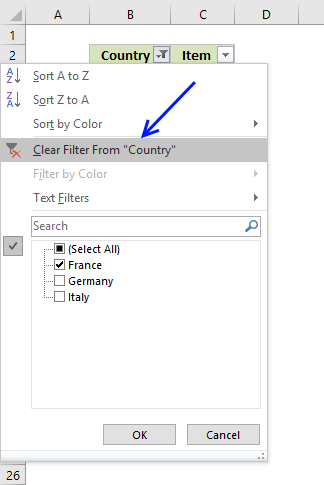3. The AutoFilter buttons next to each header are still there.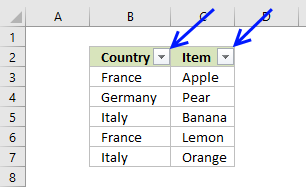4. If you want to remove those as well, go to tab "Home" on the ribbon and press with left mouse button on "Sort & Filter" button, then on "Filter"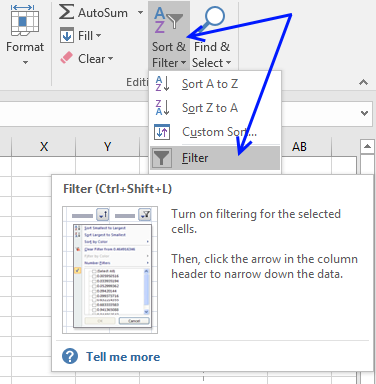5. The data set now looks like this: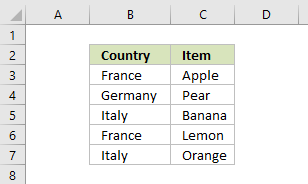## Lookup and return multiple values [Advanced Filter]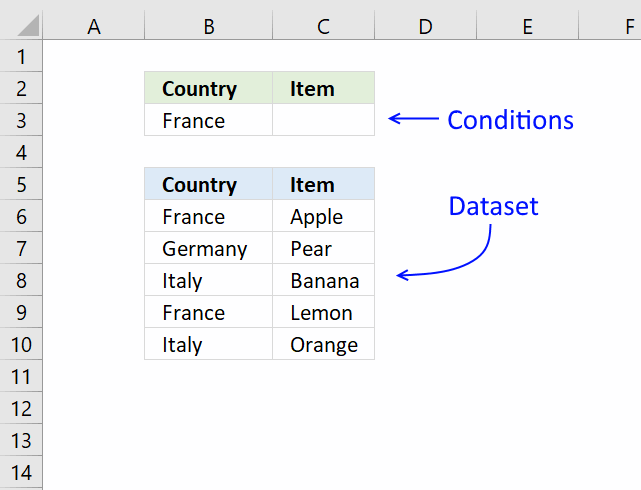The Advanced Filter is a tool in Excel that allows you to filter a dataset using complicated criteria combinations like AND - OR logic that the regular AutoFilter tool can't accomplish.

In this case I am only going to filter based on a single condition so this will be an easy introduction to the Advanced Filter in Excel.

1. Copy the dataset headers and place them above or below your dataset, this to avoid confusion if the conditions disappear when a filter is applied.
Rows will be hidden and if a condition is on the same row it will be hidden as well. I created headers on row 2, see image above.
2. Enter the condition below the correct header you want to apply a filter to, I entered my condition in cell B3.
3. Select cell range B5:C10.
4. Go to tab "Data" on the ribbon.
5. Press with left mouse button on "Advanced" button.
6. Press with left mouse button on in Criteria range: field and select cell range B2:C3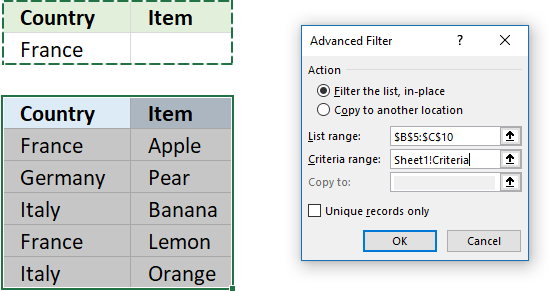7. Press with left mouse button on OK button.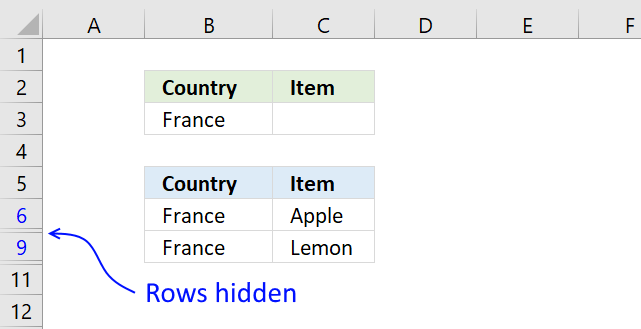The image above shows the dataset filtered based on the condition used in cell B3. To clear the filter simply go to tab "Data" on the ribbon and press with left mouse button on "Clear" button.

## Lookup and return multiple values [Excel Defined Table]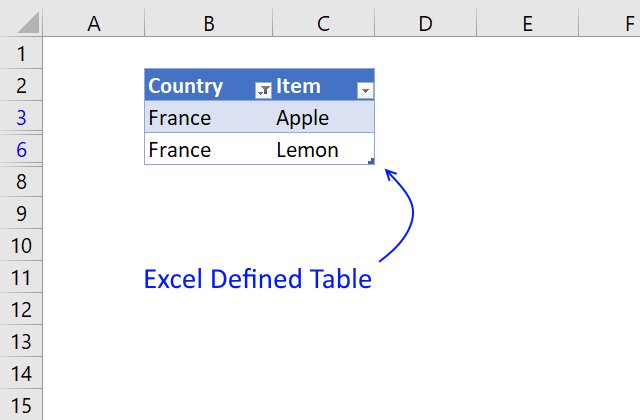The image above shows you a dataset converted to an Excel Defined Table and filtered based on item "France" in column B.

1. Select a cell in your data set.
2. Press CTRL + T (shortcut for creating an Excel Defined Table).
3. A dialog box appears, press with left mouse button on the checkbox if your data set contains headers.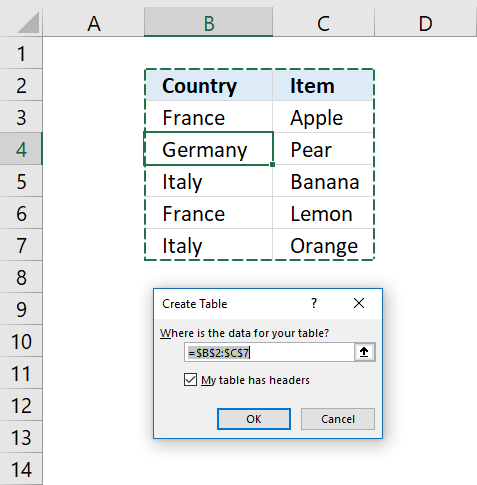4. Press with left mouse button on OK button.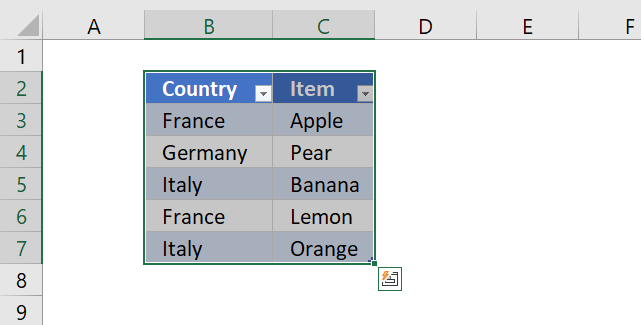To filter the table follow these simple steps:

1. Press with left mouse button on the black arrow next to a header name.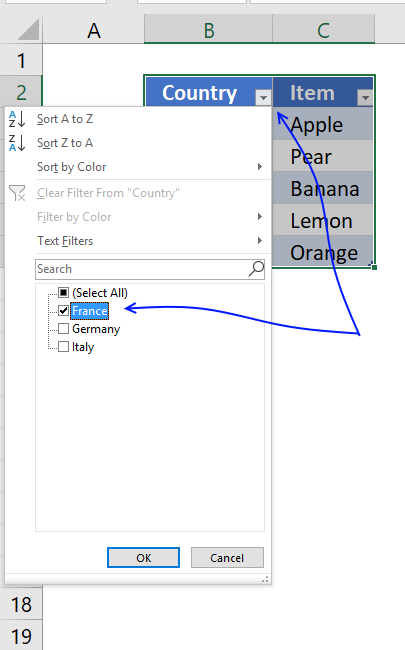2. Make sure the checkbox next to the value you want to use as a condition is selected.
3. Press with left mouse button on OK button.So why use an Excel defined Table? An Excel defined Table contains many more useful features.

• Enter a formula in one cell and Excel automatically enters the formula in the remaining Excel Table cells on the same column.
• Cell references are converted to structured references, for example a cell reference to column "Country" might look like this: Table[Country].
This is beneficial because you don't need to adjust cell references if your table grows or shrinks, the cell reference is the same no matter what. You don't need to use dynamic named ranges either.
• Easy to filter and sort data.
• Easy to add or delete data, simply type your data below the last table row and the Excel defined Table will automatically expand.
• Use as data source for a chart and the chart will display what is filtered.

## Return multiple values vertically or horizontally [UDF]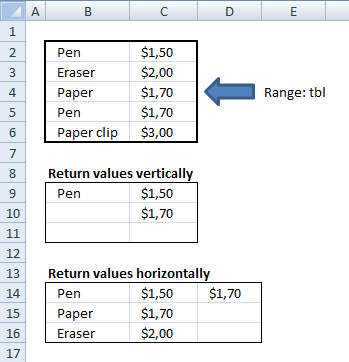Make sure you have copied the vba code below into a standard module before entering the array formula.

### User defined Function Syntax

vbaVlookup(lookup_value, table_array, col_index_num, [h])

### Arguments

 lookup_value Required. table_array Required. A cell reference to the data table you want to search. col_index_num Required. A number representing the column in the table_array. [h] Optional. Return values horizontally.

Array formula in cell C14:D14:

=vbaVlookup(B14, \$B\$2:\$C\$6, 2, "h")

### How to enter custom function array formula

1. Select cell range C9:C11
2. Type above custom function
3. Press and hold Ctrl + Shift
4. Press Enter once
5. Release all keys

A beginners guide to Excel array formulas

Array formulas allows you to do advanced calculations not possible with regular formulas.

### How to enter custom function array formula

1. Select cell range C14:D14
2. Type above custom function
3. Press and hold Ctrl + Shift
4. Press Enter once
5. Release all keys

### How to copy array formula to the next row

1. Select cell range C14:D14
2. Copy cell range
3. Select cell range C15:D15
4. Paste

### Vba code

1. Copy vba code below.
2. Press Alt + F11 to open the visual basic editor.
3. Press with right mouse button on on your workbook in the project explorer.
4. Press with mouse on "Insert".
5. Press with mouse on "Module".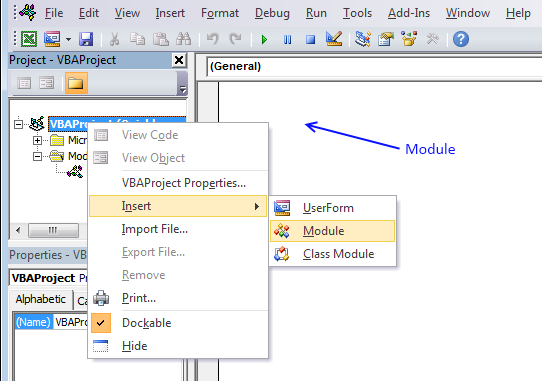6. Paste code to code module.
```'Name User Defined Function and arguments
Function vbaVlookup(lookup_value As Range, tbl As Range, col_index_num As Integer, Optional layout As String = "v")

'Declare variables and data types
Dim r As Single, Lrow, Lcol As Single, temp() As Variant

'Redimension array variable temp
ReDim temp(0)

'Iterate through cells in cell range
For r = 1 To tbl.Rows.Count

'Check if lookup_value is equal to cell value
If lookup_value = tbl.Cells(r, 1) Then

'Save cell value to array variable temp
temp(UBound(temp)) = tbl.Cells(r, col_index_num)

'Add anoher container to array variable temp
ReDim Preserve temp(UBound(temp) + 1)
End If
Next r

'Check if variable layout equals h
If layout = "h" Then

'Save the number of columns the user has entered this User Defined Function in.

'Iterate through each container in array variable temp that won't be populated
For r = UBound(temp) To Lcol

'Save a blank to array container
temp(UBound(temp)) = ""

'Increase the size of array variable temp with 1
ReDim Preserve temp(UBound(temp) + 1)
Next r

'Decrease the size of array variable temp with 1
ReDim Preserve temp(UBound(temp) - 1)

'Return values to worksheet
vbaVlookup = temp

'These lines will be rund if variable layout is not equal to h
Else

'Save the number of rows the user has entered this User Defined Function in

'Iterate through empty cells and save nothing to them in order to avoid an error being displayed
For r = UBound(temp) To Lrow
temp(UBound(temp)) = ""
ReDim Preserve temp(UBound(temp) + 1)
Next r

'Decrease the size of array variable temp with 1
ReDim Preserve temp(UBound(temp) - 1)

'Return temp variable to worksheet with values rearranged vertically
vbaVlookup = Application.Transpose(temp)
End If

End Function```